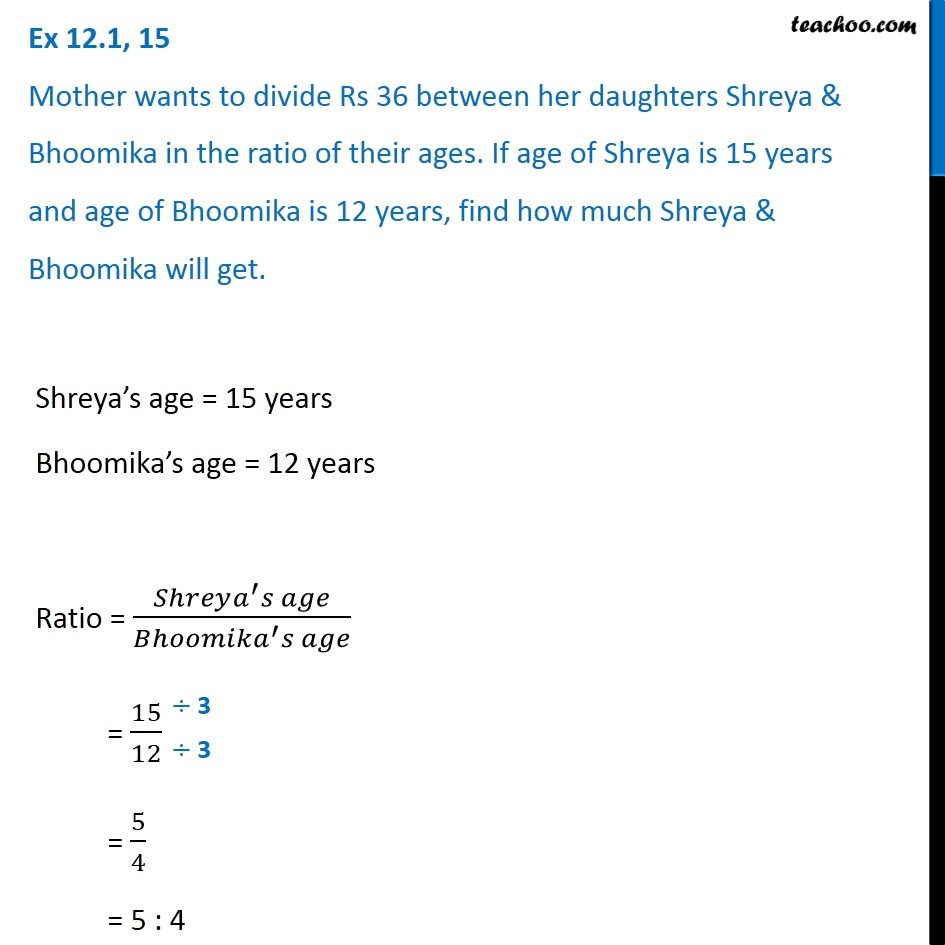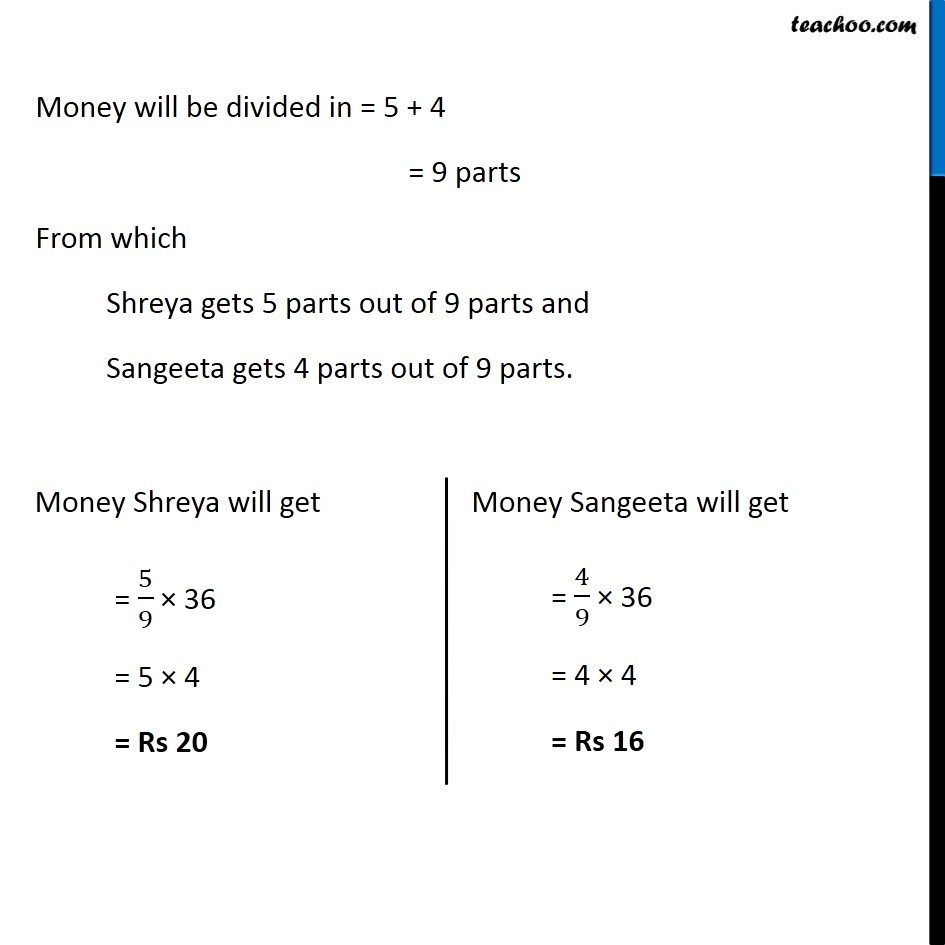Ex 12.1

Chapter 12 Class 6 Ratio and Proportion
Serial order wiseLearn in your speed, with individual attention - Teachoo Maths 1-on-1 Class

### Transcript

Ex 12.1, 15 Mother wants to divide Rs 36 between her daughters Shreya & Bhoomika in the ratio of their ages. If age of Shreya is 15 years and age of Bhoomika is 12 years, find how much Shreya & Bhoomika will get.Shreya’s age = 15 years Bhoomika’s age = 12 years Ratio = (𝑆ℎ𝑟𝑒𝑦𝑎^′ 𝑠 𝑎𝑔𝑒)/(𝐵ℎ𝑜𝑜𝑚𝑖𝑘𝑎^′ 𝑠 𝑎𝑔𝑒) = 15/12 = 5/4 = 5 : 4 Money will be divided in = 5 + 4 = 9 parts From which Shreya gets 5 parts out of 9 parts and Sangeeta gets 4 parts out of 9 parts. Money Shreya will get = 5/9 × 36 = 5 × 4 = Rs 20 Money Sangeeta will get = 4/9 × 36 = 4 × 4 = Rs 16